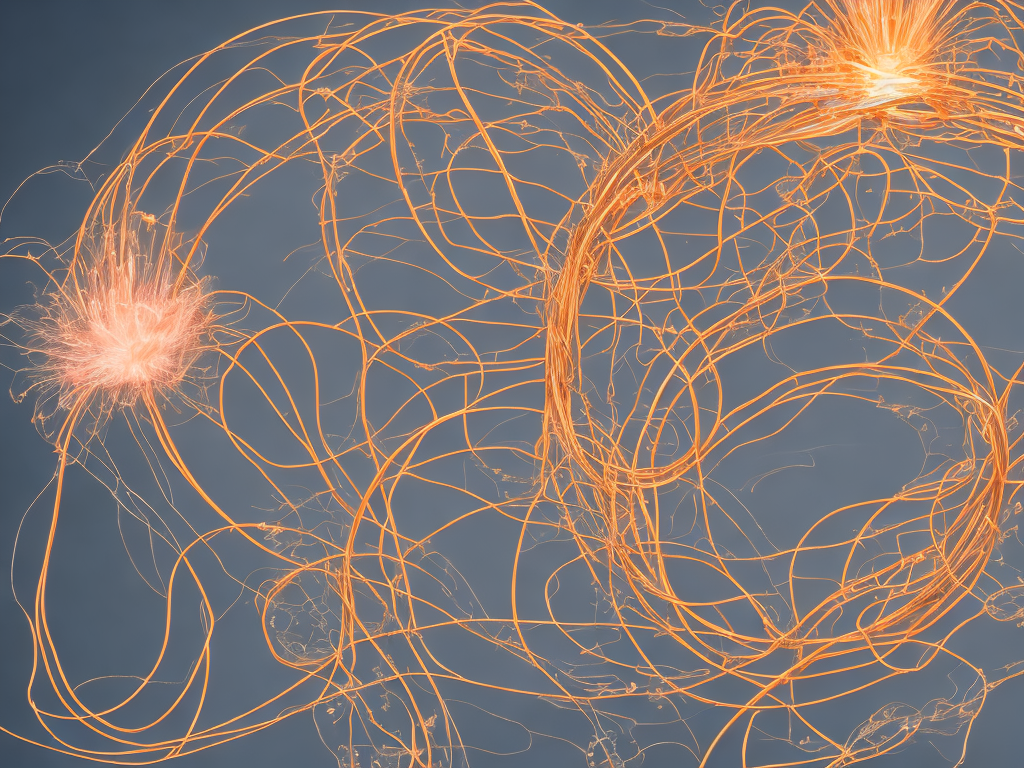# Si Unit Of Current Density

tl;dr
The SI unit of current density is amperes per square meter (A/m^2), which is derived from the base unit of electric current, the ampere (A), and describes the flow of electric charge through a medium per unit area.The concept of current density is an important one in electrical engineering and physics, as it describes the flow of electric charge in a medium, particularly through a solid conductor. In order to quantify this flow, the SI unit of current density was created.

Current density is defined as the flow of charge per unit area, and is typically represented by the symbol J. It is important to note that current density is a vector quantity, meaning it has both magnitude and direction. This is because it is related to the flow of charge through a surface, which will depend on the orientation and geometry of that surface.

The SI unit of current density is amperes per square meter, or A/m^2. This unit is derived from the base unit of electric current, the ampere (A), which is defined as the amount of charge passing through a conductor per unit time. The square meter component reflects the fact that current density is a measure of charge flow per unit area.

To give an example of how current density might be used, consider a simple circuit consisting of a battery and a wire. If we want to understand the flow of electric charge through the wire, we might use the concept of current density. Suppose that the wire has a cross-sectional area of 1 square millimeter (mm^2), and that the current passing through it is 1 ampere. Then the current density would be:

J = I/A

J = 1 A / (0.001 m^2) = 1000 A/m^2

This calculation tells us that the current through the wire is relatively high, as the current density is large. However, it is important to keep in mind that current density is not the only factor that determines the behavior of electric circuits; other factors such as voltage drop, resistance, and capacitance also play important roles.

One application of current density is in the design of electrical conductors (such as wires) that can carry high current densities without overheating or otherwise failing. This is important in many areas of engineering, including power transmission and distribution, motor design, and electronics. When designing conductors, it is necessary to calculate the expected current density under normal operating conditions and ensure that the conductor is capable of handling this level of current without excessive heating or damage.

In addition to the SI unit of current density, there are other units that are sometimes used in specific contexts. For example, in electrochemistry, current density is sometimes expressed in units of milliamperes per square centimeter (mA/cm^2), while in semiconductor physics it is often given in units of amperes per square centimeter (A/cm^2). These units are simply scaled versions of the SI unit, and represent the same basic concept of charge flow per unit area.

Overall, the SI unit of current density provides an important tool for understanding the behavior of electrical circuits and designing electrical components. Its use can help to ensure that electrical systems operate safely and efficiently, and can contribute to advances in fields such as renewable energy, transportation, and communications.